• 时钟样式】男神数字，女神数字，梵高数字，王思聪吃热狗数字，手势数字，大写数字，中文汉字，麻将数字，扑克数字。 电脑壁纸下载可在wallpaper engine或者upupoo上搜索全称“冷高轮时间‘ 电脑屏保可在冷高轮时间...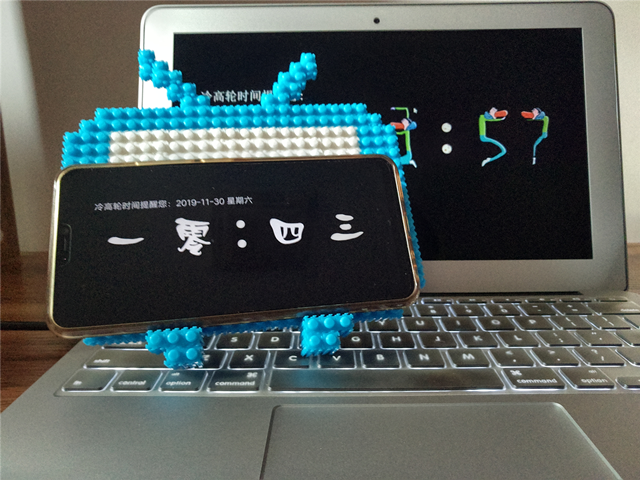【应用名称】:冷高轮时间
【应用大小】：6.83
【支持平台】：安卓(Android)，苹果（iOS）
【时钟样式】男神数字，女神数字，梵高数字，王思聪吃热狗数字，手势数字，大写数字，中文汉字，麻将数字，扑克数字。
电脑壁纸下载可在wallpaper engine或者upupoo上搜索全称“冷高轮时间‘
电脑屏保可在冷高轮时间官网下载：lenggaolun。com（com前面是点不是句号)
手机APP可在android和ios各大应用市场搜索全称’冷高轮时间“下载
【软件介绍】：闲置手机，旧手机发光发亮
适合任何场景使用，办公桌，卧室床头柜，自习考研，学校寝室，，，，，显示，逼格高。
功能丰富，时间显示年/月/日 星期 秒表 闹钟 简单干净，时间类功能较为丰富。
应用描述：
全屏显示 占用内存小 精致美观 界面干净 创意元素新颖
精确到秒
功能：
-手电筒
-时/分/秒显示
-年/月/日/星期
-秒表 闹钟
-12小时和24小时切换
-亮度调节
-时钟样式切换
-隐藏秒
-防烧屏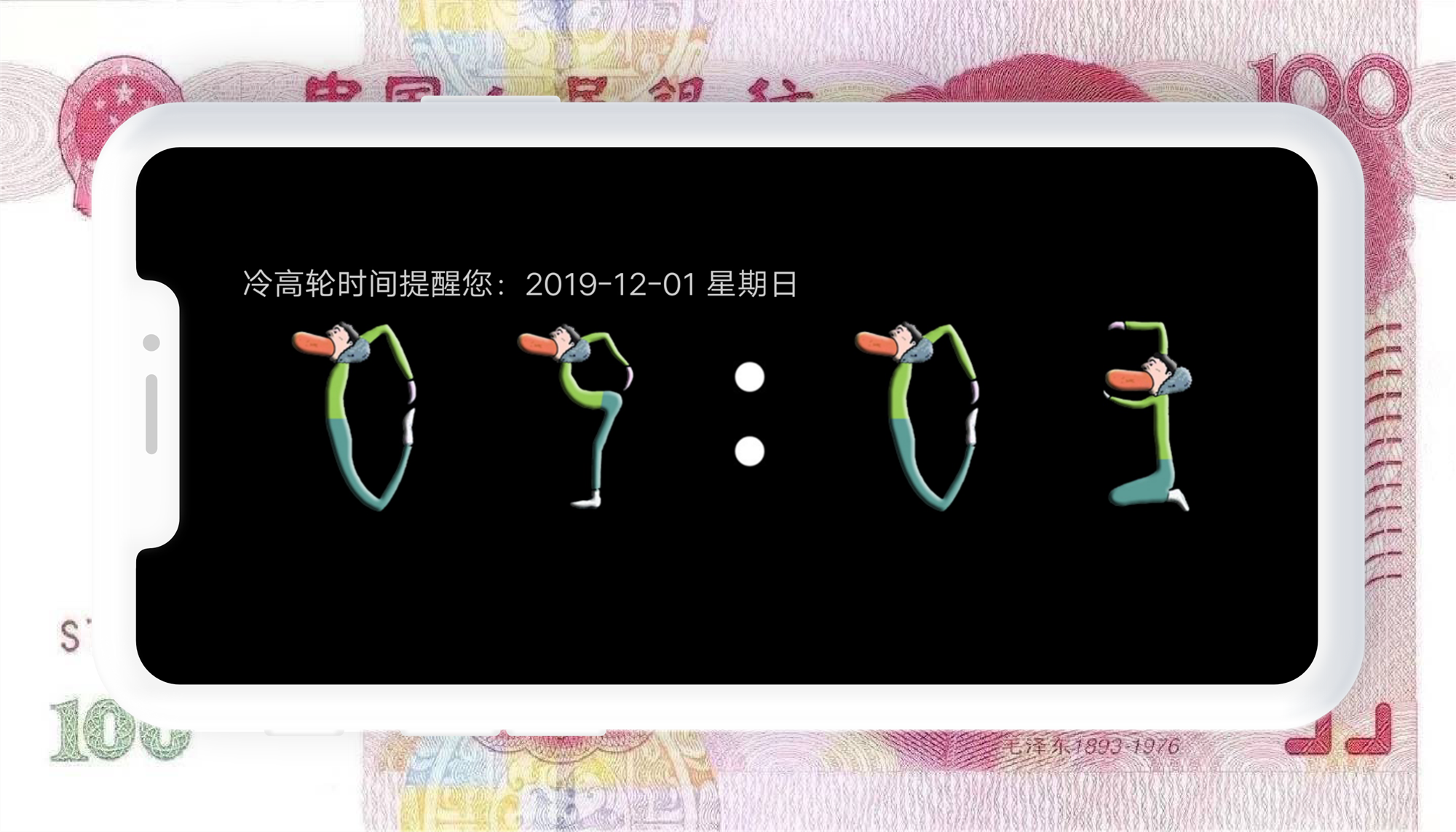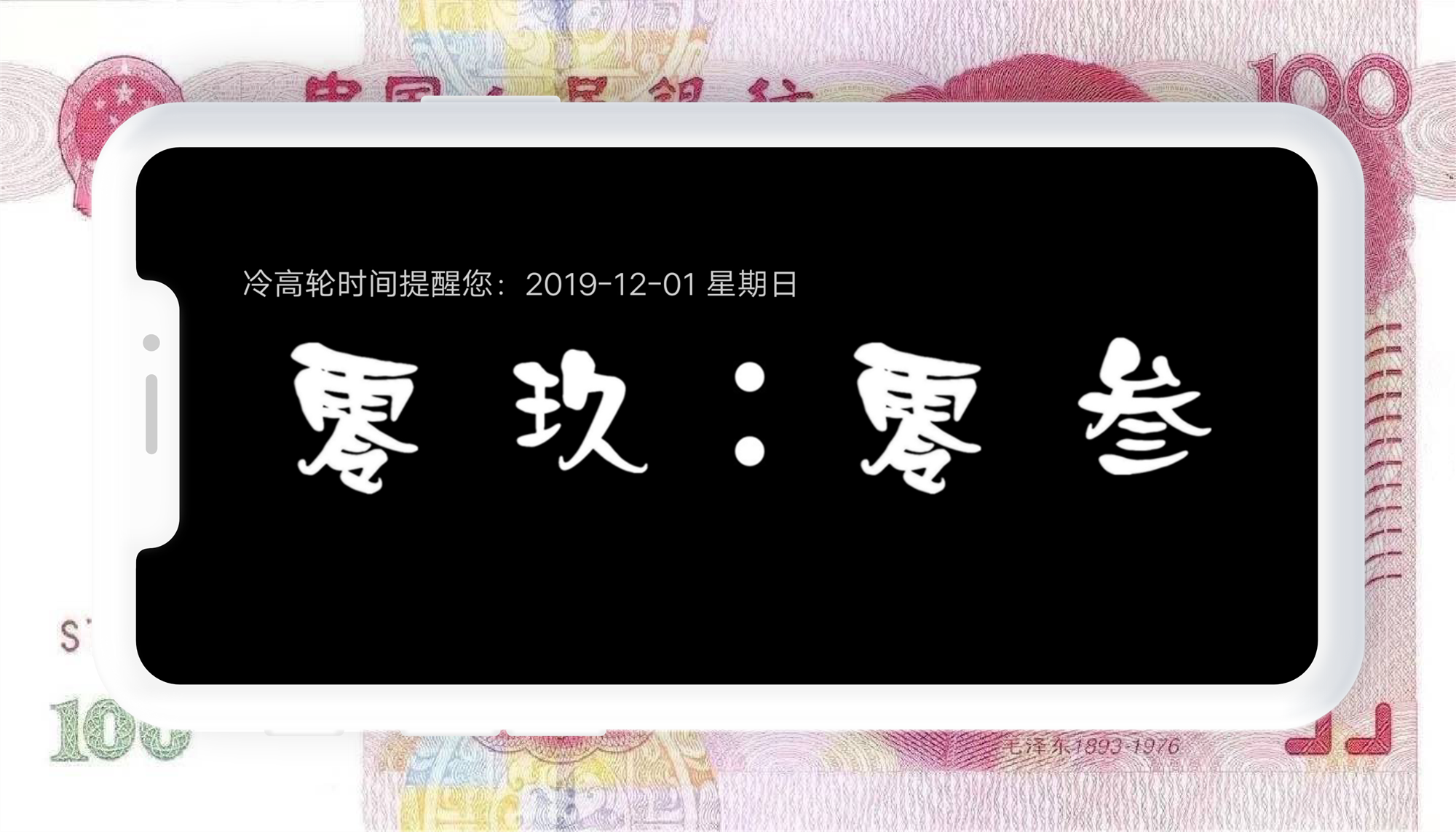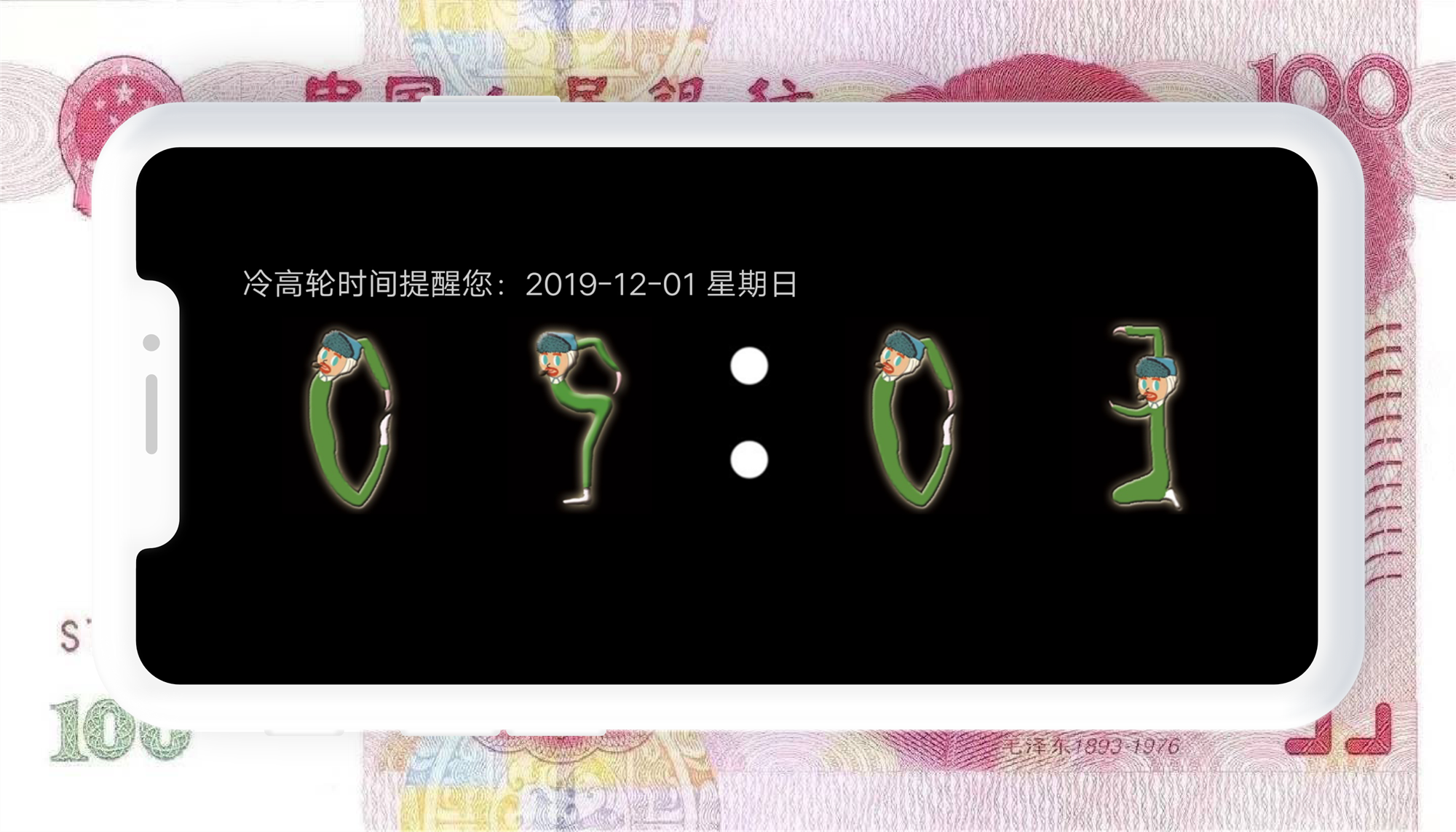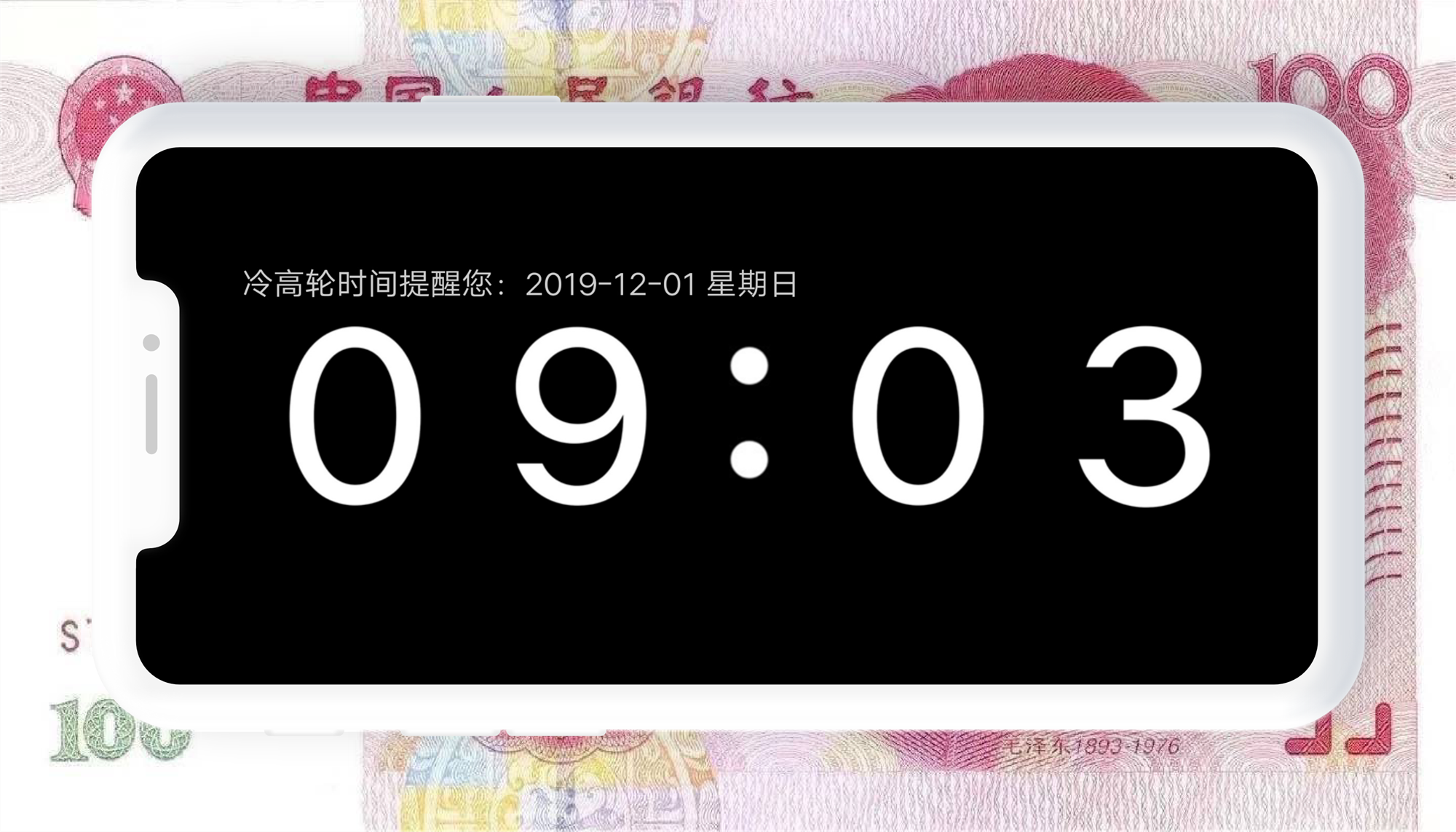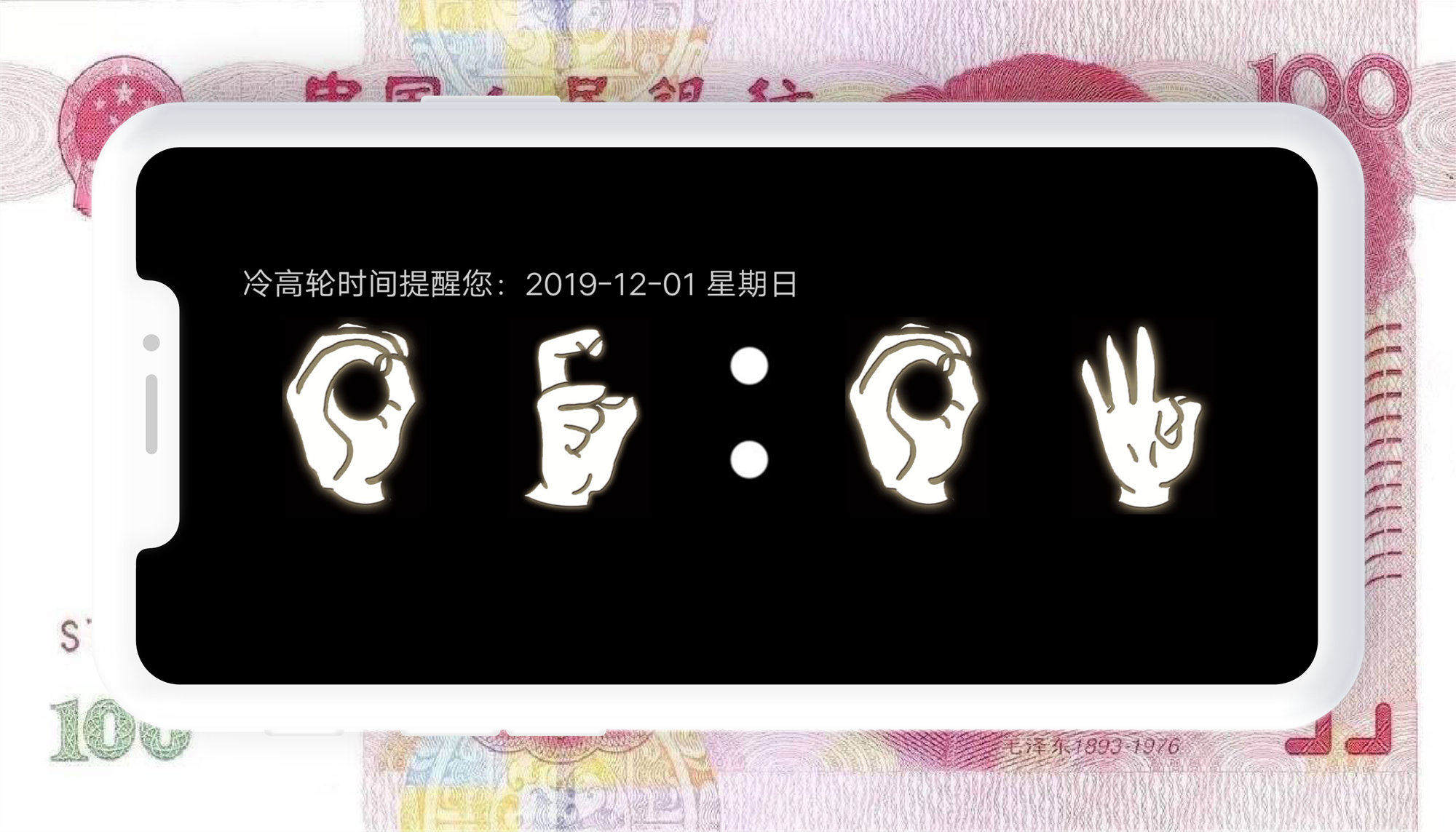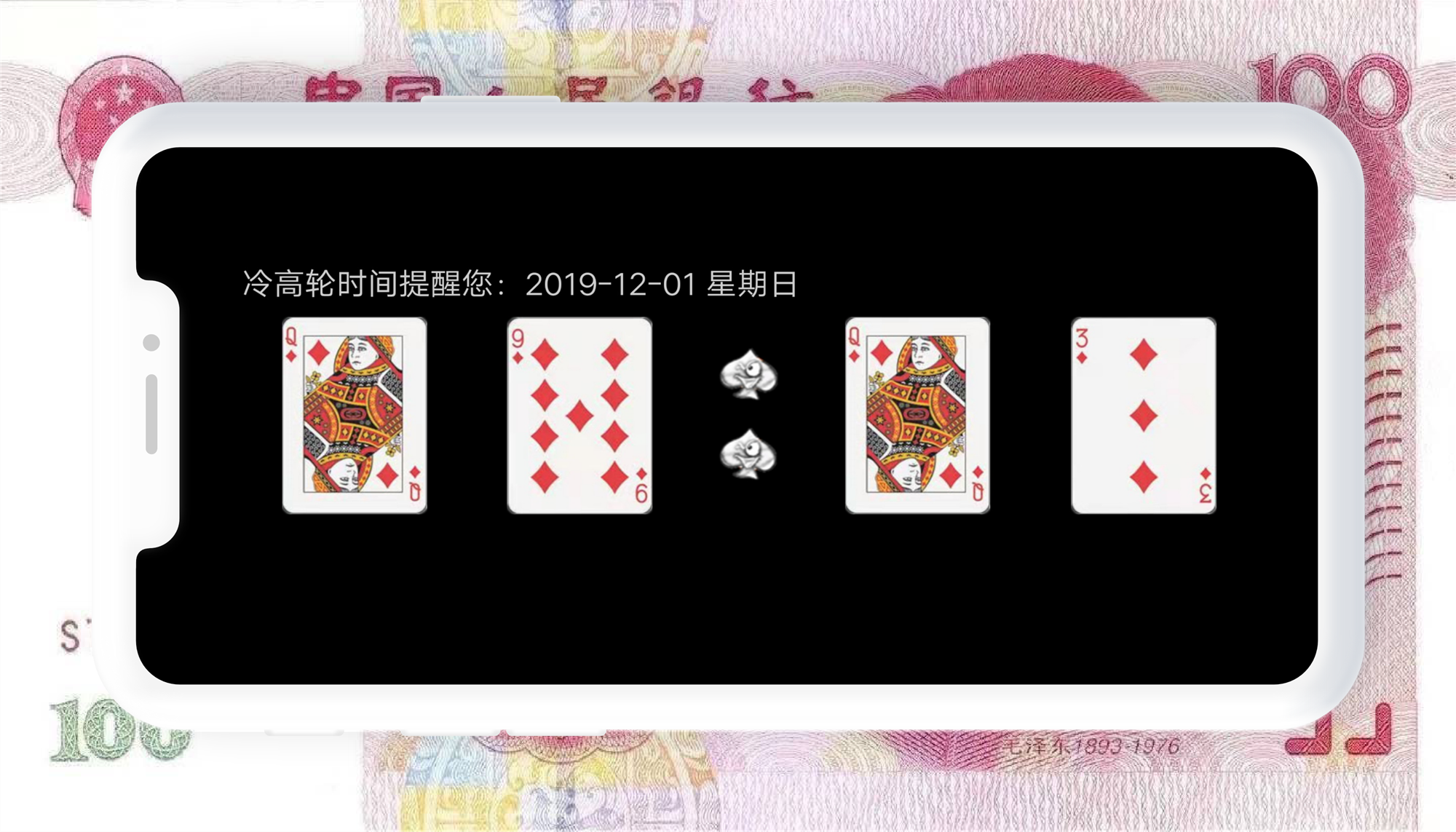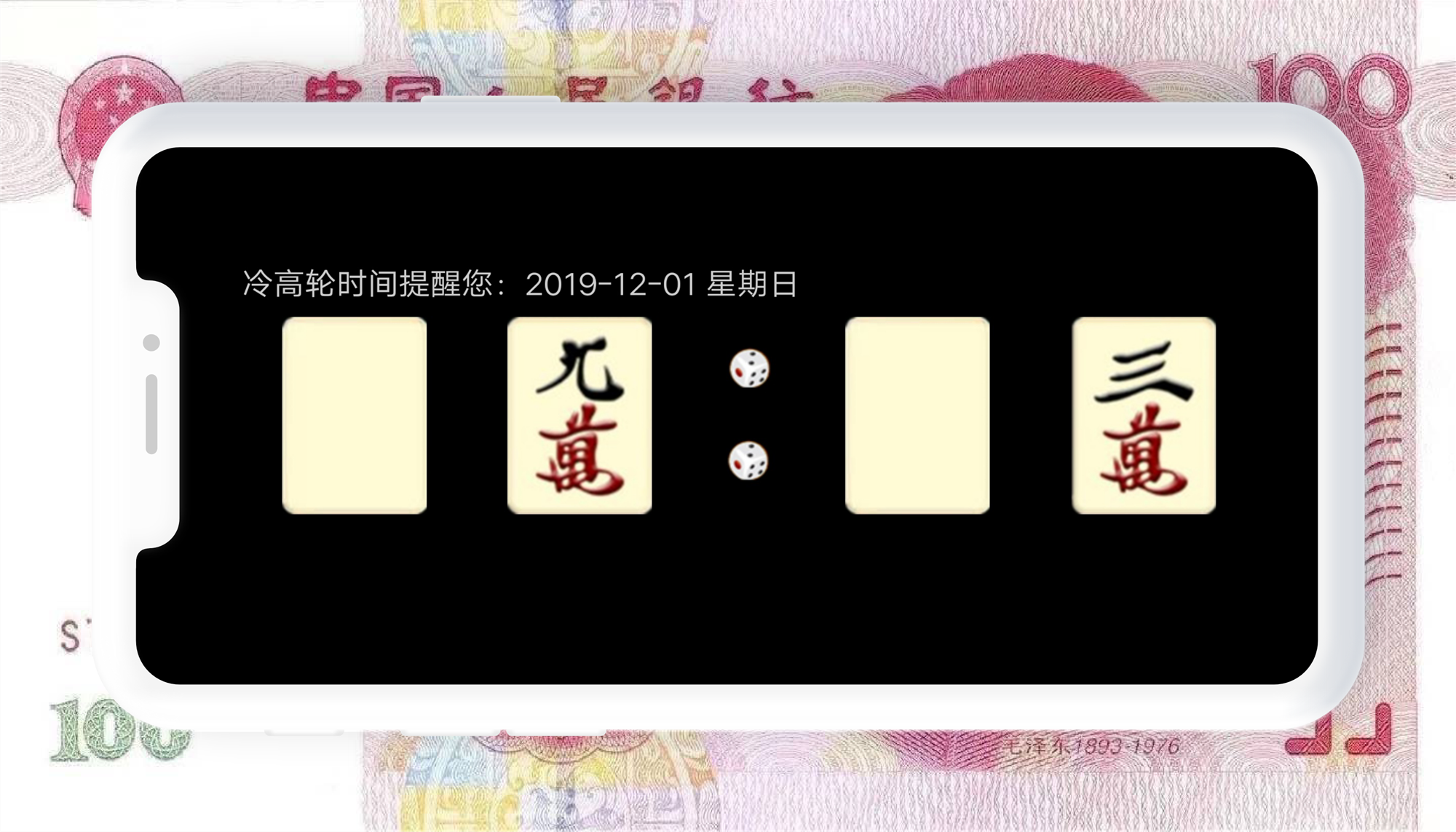展开全文• 摘要:VB源码,系统相关,数字时钟,电子　VB图像数字时钟，VB源码下载，数字电子时钟，显示时间和日期，惯用的风格，实用性强，源码供大家下载学习。
• ## 数字时钟字体下载

热门讨论 2012-07-14 10:37:26
把字体放在 C:\WINDOWS\Fonts下即可 查看效果，复制到地址栏就可以看到图片 http://c.hiphotos.baidu.com/space/pic/item/d50735fae6cd7b896c1afd130f2442a7d9330e28.jpg
• 数字时钟_VHDL程序 数字时钟_VHDL程序 数字时钟_VHDL程序
• ## 数字时钟

千次阅读 2014-07-16 21:32:07
这是一个数字钟表程序，主要功能是从系统中获取当前的系统时间然后再将其显示在数字时钟上，由于整个的数字时钟都是用函数构成的，所以它可以实现一般的数字时钟所不具有的功能，比如说它可以被鼠标指针拖动到窗口...

软件简介：

这是一个数字钟表程序，主要功能是从系统中获取当前的系统时间然后再将其显示在数字时钟上，由于整个的数字时钟都是用函数构成的，所以它可以实现一般的数字时钟所不具有的功能，比如说它可以被鼠标指针拖动到窗口的任意位置，除此之外它还可以实现钟表大小随鼠标滚轮的滚动而变大变小的操作。

源代码：

package TheClock;
import java.awt.*;
import javax.swing.*;
import java.lang.*;
import java.awt.event.*;
import java.util.*;
public class TheClock extends JApplet implements Runnable,MouseMotionListener,MouseListener,MouseWheelListener{
boolean dstatus=true;
public static final double PI=Math.PI/180;
Image offScreenImage=null;
Graphics offScreenBuffer=null;
int width=1440;
int height=900;
double R=90;
int
cx=250,cy=150;
int x2,y2;
public void init(){
t.start();
offScreenImage=this.createImage(width,height);
offScreenBuffer=offScreenImage.getGraphics();
}
public void update(Graphics g){
paint(g);
}
public void mouseClicked(MouseEvent e){}
public void mousePressed(MouseEvent e){}
public void mouseReleased(MouseEvent e){}
public void mouseEntered(MouseEvent e){}
public void mouseExited(MouseEvent e){}
public void mouseDragged(MouseEvent e){
x2=e.getX();
y2=e.getY();
cx=x2;
cy=y2;
repaint();
}
public void mouseMoved(MouseEvent e){}
public void mouseWheelMoved(MouseWheelEvent e){
int count=e.getWheelRotation();
if(count>0){
R+=count;
}else if(count<0){
R+=count;
}
}
public void run(){
while(true){
try{
t.sleep(1000);
}catch(Exception e){}
repaint();
}
}
public void paint(Graphics g){
int s,m,h;
//创建时间
Date rightNow=new Date();
//获取系统时间
String today=rightNow.toLocaleString();
s=rightNow.getSeconds();
m=rightNow.getMinutes();
h=rightNow.getHours();
g.drawImage(offScreenImage,0,0,this);
g.setColor(Color.orange);
g.fillOval((int)(cx-R), (int)(cy-R),(int)(2*R),(int)(2*R));
g.setColor(Color.black);
g.drawOval((int)(cx-R), (int)(cy-R),(int)(2*R),(int)(2*R));
//画刻度（1,2,4,5,7,8,10,11）

g.drawString("12", cx-8, (int)(cy-R+18));
g.drawString("3", (int)(cx+R-18), cy+4);
g.drawString("6",cx-4,(int)(cy+R-10));
g.drawString("9", (int)(cx-R+12), cy+4);
g.drawString("我的钟表", cx-20, (int)(cy-R-20));
g.drawString(today,cx-55,(int)(cy+R+30));
g.setFont(new Font("TimesRoman",Font.PLAIN,14));
offScreenBuffer.clearRect(0,0,width,height);
drawCircle(g,cx,cy,R,s,m,h);
}
public void drawCircle(Graphics g,double x,double y,double R,double s,double m,double h){
double x1,y1;
x1=x+R*Math.cos((s*6-90)*PI);
y1=y+R*Math.sin((s*6-90)*PI);
//画秒针
g.setColor(Color.blue);
g.drawLine((int)x, (int)y, (int)x1, (int)y1);
//画分针
g.setColor(Color.green);
g.drawLine((int)x, (int)y, (int)(x+(R/4*3)*Math.cos((m*6-90)*PI)), (int)(y+(R/4*3)*Math.sin((m*6-90)*PI)));
//画时针
g.setColor(Color.red);
g.drawLine((int)x, (int)y, (int)(x+(R/2)*Math.cos((h*30+m*6/12-90)*PI)), (int)(y+(R/2)*Math.sin((h*30+m*6/12-90)*PI)));
//画刻度（3,6,9,12）
g.setColor(Color.black);
g.drawLine((int)x,(int)(y-R+10),(int)x,(int)(y-R));
g.drawLine((int)(x+R-10),(int)y,(int)(x+R),(int)y);
g.drawLine((int)x,(int)(y+R-10),(int)x,(int)(y+R));
g.drawLine((int)(x-R+10),(int)y,(int)(x-R),(int)y);
g.drawLine((int)(x+(R-5)*Math.cos(30*PI)),(int)(y+(R-5)*Math.sin(30*PI)),(int)(x+R*Math.cos(30*PI)),(int)(y+R*Math.sin(30*PI)));
g.drawLine((int)(x+(R-5)*Math.cos(60*PI)),(int)(y+(R-5)*Math.sin(60*PI)),(int)(x+R*Math.cos(60*PI)),(int)(y+R*Math.sin(60*PI)));
g.drawLine((int)(x+(R-5)*Math.cos(120*PI)),(int)(y+(R-5)*Math.sin(120*PI)),(int)(x+R*Math.cos(120*PI)),(int)(y+R*Math.sin(120*PI)));
g.drawLine((int)(x+(R-5)*Math.cos(150*PI)),(int)(y+(R-5)*Math.sin(150*PI)),(int)(x+R*Math.cos(150*PI)),(int)(y+R*Math.sin(150*PI)));
g.drawLine((int)(x+(R-5)*Math.cos(210*PI)),(int)(y+(R-5)*Math.sin(210*PI)),(int)(x+R*Math.cos(210*PI)),(int)(y+R*Math.sin(210*PI)));
g.drawLine((int)(x+(R-5)*Math.cos(240*PI)),(int)(y+(R-5)*Math.sin(240*PI)),(int)(x+R*Math.cos(240*PI)),(int)(y+R*Math.sin(240*PI)));
g.drawLine((int)(x+(R-5)*Math.cos(300*PI)),(int)(y+(R-5)*Math.sin(300*PI)),(int)(x+R*Math.cos(300*PI)),(int)(y+R*Math.sin(300*PI)));
g.drawLine((int)(x+(R-5)*Math.cos(330*PI)),(int)(y+(R-5)*Math.sin(330*PI)),(int)(x+R*Math.cos(330*PI)),(int)(y+R*Math.sin(330*PI)));
}
}

运行的结果：展开全文源代码
• Proteus C源码 数字时钟 可调时间 Proteus C源码 数字时钟 可调时间 Proteus C源码 数字时钟 可调时间
• 壁纸名称：冷高轮时间大写数字时钟 壁纸样式：梵高数字动态时钟，王思聪吃热狗数字动态时钟，手势数字动态时钟，大写数字动态时钟，中文汉字动态时钟，麻将数字动态时钟，扑克数字动态时钟 电脑壁纸下载可在...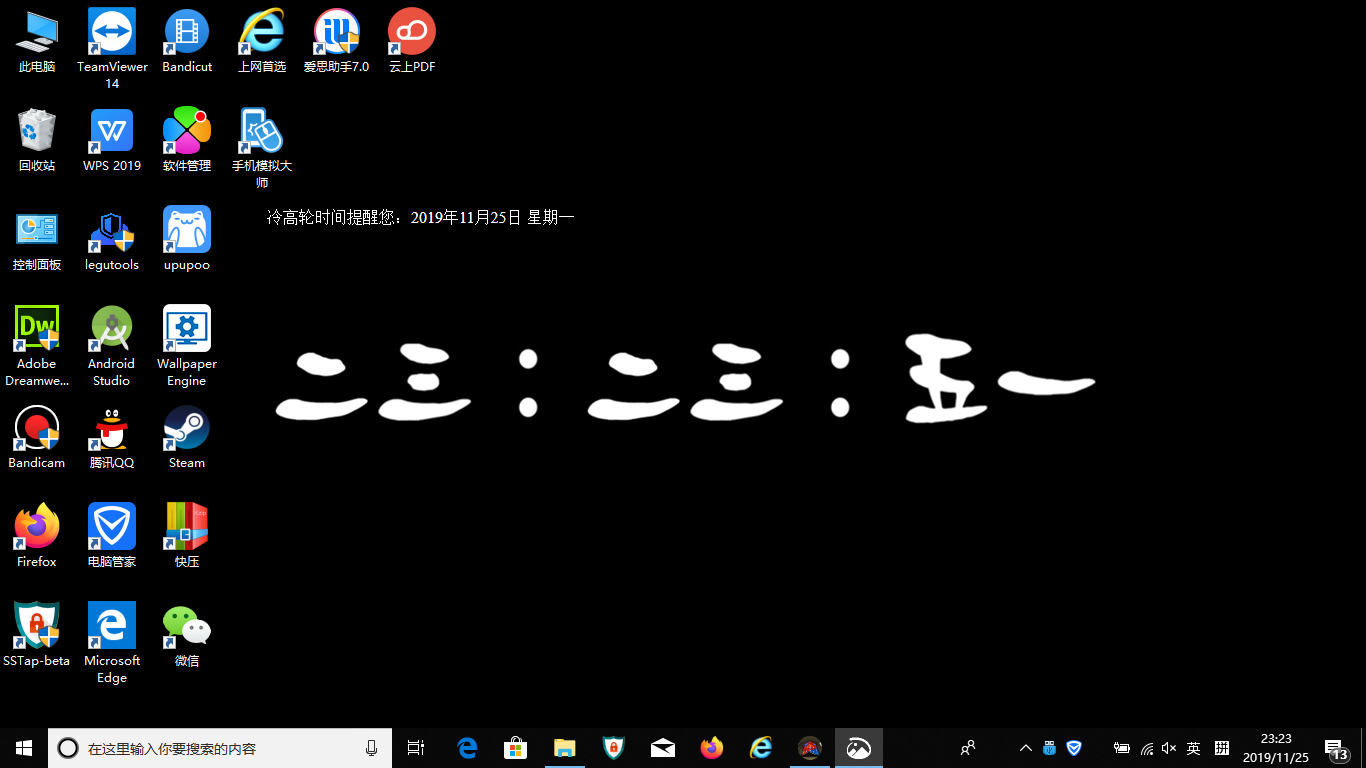壁纸名称：冷高轮时间
壁纸样式：梵高数字动态时钟，王思聪吃热狗数字动态时钟，手势数字动态时钟，大写数字动态时钟，中文汉字动态时钟，麻将数字动态时钟，扑克数字动态时钟
电脑壁纸下载可在wallpaper engine或者upupoo上搜索全称“冷高轮时间‘
电脑屏保可在冷高轮时间官网下载：lenggaolun。com（com前面是点不是句号)
手机APP可在android和ios各大应用市场搜索全称’冷高轮时间“下载。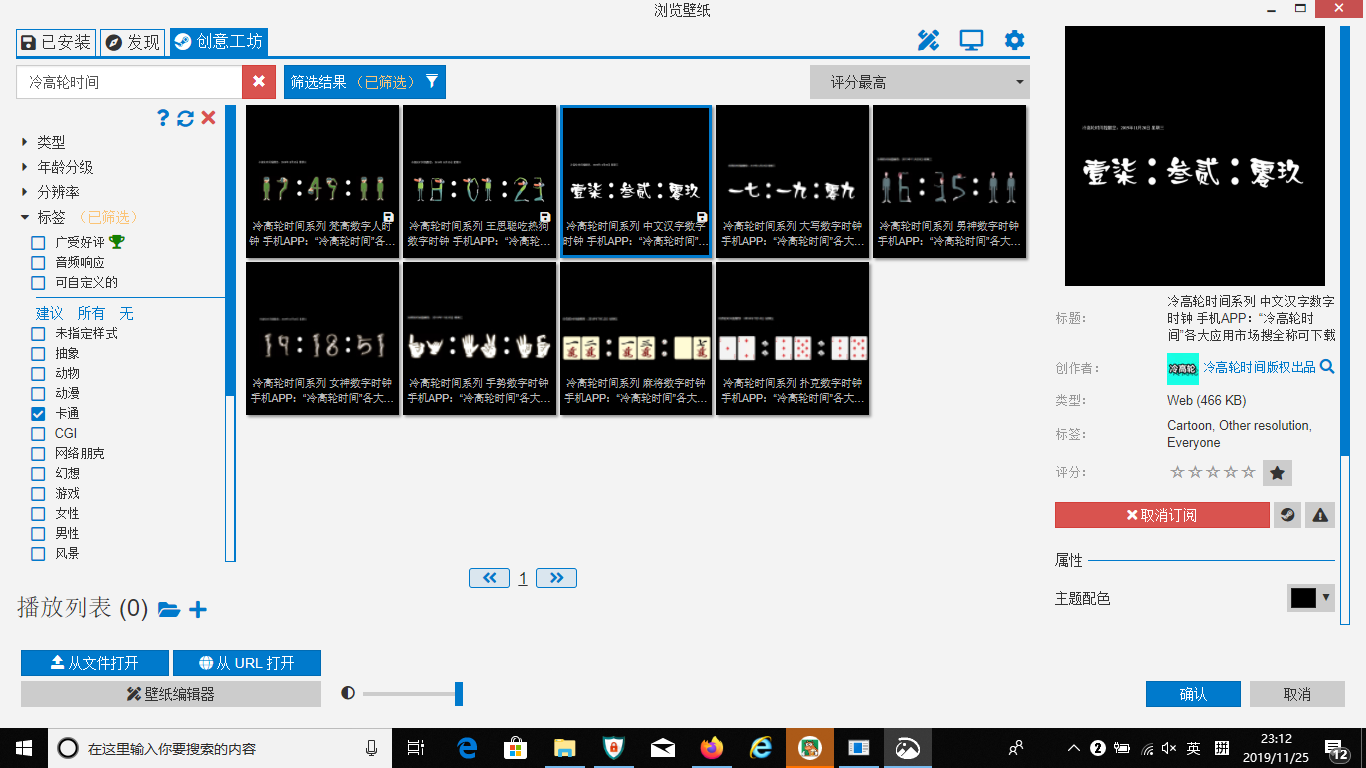展开全文• 软件名称：冷高轮时间大写数字时钟屏保 软件大小：752KB 软件语言：简体中文 软件类别：桌面工具 软件授权：免费软件 适用平台：WinXP, Win7, Win8, Win10, WinAll 电脑壁纸下载可在wallpaper engine或者upupoo上...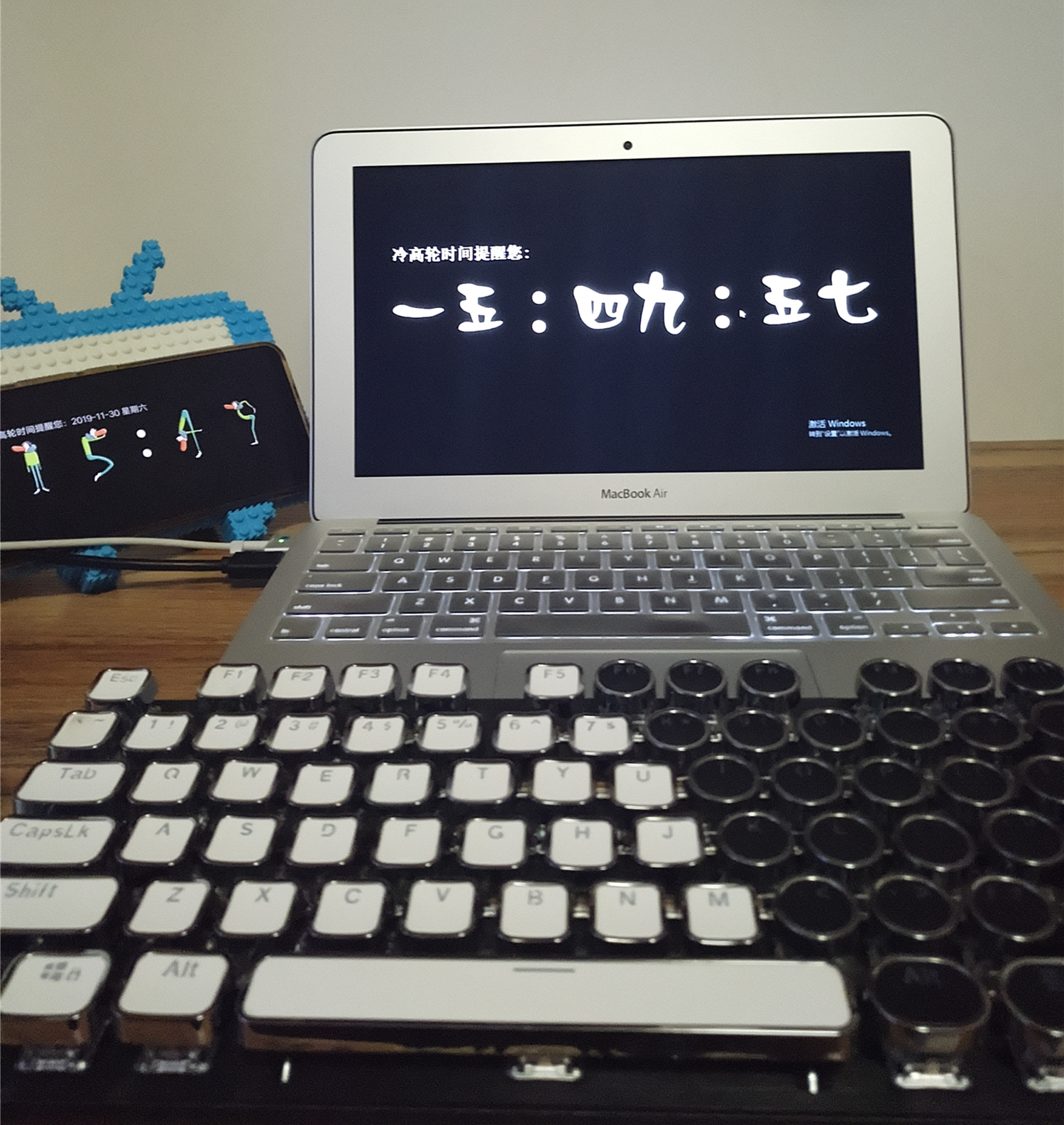软件名称：冷高轮时间大写数字时钟屏保
软件大小：752KB
软件语言：简体中文
软件类别：桌面工具
软件授权：免费软件
适用平台：WinXP, Win7, Win8, Win10, WinAll
电脑壁纸下载可在wallpaper engine或者upupoo上搜索全称“冷高轮时间‘
电脑屏保可在冷高轮时间官网下载：lenggaolun。com（com前面是点不是句号)
手机APP可在android和ios各大应用市场搜索全称’冷高轮时间“下载。
【时钟样式】男神数字，女神数字，梵高数字，王思聪吃热狗数字，手势数字，大写数字，中文汉字，麻将数字，扑克数字。


展开全文• 制作一个数字时钟标签组件，显示当前时间，并将该标签添加到框架中。java swing
• 一个简易数字时钟，由两个六十进制和一个二十四进制组成
• 课程设计的数字时钟的课设，设计非常详细，有电路图仿真的电路设计，按着上面的连线就行了，用了LS90在proteus上能出现结果，而且做出是实物，真的花了不少的心血和努力！
• 数字时钟是一种用数字电路技术实现时、分、秒计时的装置，与机械式时钟相 比具有更高的准确性和直观性，且无机械装置，具有更长的使用寿命，数字时钟可 植入自动控制、测试等系统内部，作为系统的时钟源，可为系统...
• 压缩包中的文件可以直接打开进行运行，时钟包括年月日，时钟可以自己动，也可以自己调时间，也可以暂停，没有分闰年平年。
• ewb设计数字时钟，基于数字电子技术实验的仿真电路，
• ## FPGA数字时钟计数器

千次阅读 2018-11-08 17:58:47
FPGA数字时钟计数器 好久没有登博客了,周末出去浪了一转！！！ 今天给大家讲一下数字时钟计数器，上个月写的。只是简单的数字时钟计数，没有设置闹钟、设置时间和秒表的功能，下次我会发一个带有功能的数字时钟，...
• 数字时钟是一款jquery css3实现的数字时钟特效。
• 数字时钟 设计一个能显示日期、小时、分钟、秒的数字电子，并具有整点报时的功能。 由晶振电路产生1HZ标准的信号。分、秒为六十进制计数器，时为二十四进制计数器。 可手动校正时、分时间和日期值。
• ## 数字时钟设计

万次阅读 多人点赞 2017-12-08 21:00:15
设计一个多功能数字时钟，有基本的数字时钟、秒表、闹钟三个功能。FPGA
• 数字时钟不是数字的始祖 的的的的的的ddddddd、
•字体
• 数字时钟 数字时钟数字时钟数字时钟数字时钟数字时钟数字时钟数字时钟数字时钟数字时钟数字时钟数字时钟数字时钟
• ## 数字时钟位图资源

热门讨论 2013-07-24 12:23:54
数字时钟的位图资源 (主要用于我的博文的位图资源下载)
• 数字逻辑课程设计-数字时钟 课程设计的三个简单要求 1、设计一个能显示日期、小时、分钟、秒的数字电子，并具有整点报时的功能。 2、由电路产生1HZ标准的信号。分、秒为六十进制计数器，时为二十四进制计数器。 3...
• jQuery数字时钟插件MyDigitClock是一款可以在网页上加个简单的数字钟，还有一点动画效果，你可以指定颜色字型，有没有跳动效果等，支持一页显示多个钟。
• 数字时钟实验报告，数字时钟可以实现计时，整点报时，校正时钟功能。
• 数字钟是一种用数字电路技术实现时、分、秒计时的装置，与机械式时钟相比具有更高的准确性和直观性，且无机械装置，具有更更长的使用寿命，因此得到了广泛的使用。 数字钟从原理上讲是一种典型的数字电路，其中包括......